IF YOU CAN SEE THIS go to /sfa/Nuprl/Shared/Xindentation_hack_doc.html

### Extracting Witnesses from Proofs

As explained in Sequents with Type Conclusions, when the conclusion of a sequent is a type expression, the sequent is construed as claiming the existence of a "witness" expression. In the simple case of a sequent having no hypotheses or declarations, such as "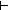T", it simply claims the type to have a member "t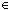T". The use of these type-conclusioned sequents permits us forestall the explicit production of such witness expressions, sometimes completely, in a proof. And yet in Nuprl, when the proof is completed, one may "extract" from the proof such a witness expression.

For example, from the proof named "sfa_doc_find_intlist_great_bound" given for Thm* x:(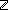List)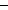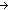{y:y greater-bounds x }, which claims the existence of a function finding an integer bigger than all members of an integer list, the Nuprl system extracts: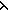x.Case of x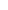x.Canil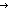-3x.Cau.v, rec:%(%1.(%3.InjCase(%3%%1%4u+2))x.Cau.v, rec:%(%1.((%3.%3(u,%1))(ext{decidable__lt})))x.Cau.v, rec:%(%)

which is Computationally Equivalent, after a a bit of simplification and variable renaming, to the expressionx.Case of xx.Canil-3x.Cau.v, rec:wInjCase(if u<winl(*) ; inr(a.*) fi; bwcu+2)

The term ext{decidable__lt} was defined by the system to expand to the term extracted from the theorem named "decidable__lt", which was used as a lemma in the proof sfa_doc_find_intlist_great_bound, and expanded toi,j. if i<jinl(*) ; inr(.*) fi. Indeed, there is also a term ext{sfa_doc_find_intlist_great_bound} which expands in one step to the proof extract, which is how I found the above extract expression for this proof in the first place.

Thm* ext{sfa_doc_find_intlist_great_bound}
Thm*x:(List){y:y greater-bounds x }

ext{sfa_doc_find_intlist_great_bound}([1; 4; 2; -1]) *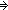6

ext{sfa_doc_find_intlist_great_bound}([1; 2; -1]) *4

ext{sfa_doc_find_intlist_great_bound}(nil) *-3

The reason the system is able to find such witnesses to extract is that each rule for inferring a type-conclusioned sequent stipulates how to build a witness, perhaps using witnesses from proofs of its subgoals.

Let's look at another example. In Sequents with Restricted Declarations there was discussion of sequents with variables barred from use in witnesses. The extracts from the theorems contrasted there are:

Thm* (k,xk)
Thm* =
Thm* ext{sfa_doc_find_greater_bound_stupidly}
Thm*k: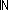x:(k List){y:(k+1)| y greater-bounds x }

Thm* (x.Case of x
Thm* (x.Canil0
Thm* (x.Cau.v, rec:rInjCase(if u<rinl(*) ; inr(.*) fi; arbu+1))
Thm* =
Thm* ext{sfa_doc_find_small_greater_bound}
Thm*(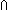k:x:(k List){y:(k+1)| y greater-bounds x })

Representing Propositions as Types enables Propositional Proof Witness Extraction of constructive content.

(March 2001 - sfa )